## Friday, September 27, 2013

Consider an urn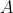, with 15 blue balls, and 10 red balls, and an urn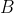, with 10 blue balls, and 15 red balls. We select randomly one urn (with probability 50% for each urn).
We draw a ball, which turns out to be blue, and we put it back in the urn, Now, we draw a (second) ball. What is the probability that this (second) ball is blue?

Solution after the break!

The question is here to compute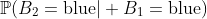and according to Bayes formula, it is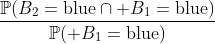Now, to compute those two probabilities, we have to condition on the urn,Given the urn, since we replace the ball,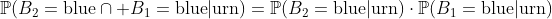i.e.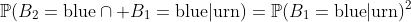So if we substitute numerical probabilities to get a blue ball in the previous formula, we get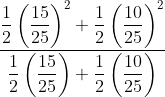which not the same as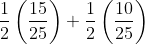Here, we get
`0.52 `

By : http://freakonometrics.hypotheses.org/8718

-->